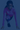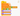Code ChampionshipBasic If Logic

Code Championship Puzzle - 2019-06-06

Difficulty: Level 3

Can you solve this puzzle? What is the Answer?

Take a look at the code below and see if you can find the answer! What is the Answer that the computer will have at the end of these lines of code? When you think you have the answer. Click the "Show Answer" button!

In this puzzle, check out the two variables and what the computer does with them. Figure out what the answer is, and then figure out how the if block works.If you liked this puzzle, you should check out the other things we do!